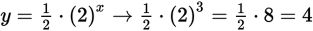# SAT Math Multiple Choice Question 849: Answer and Explanation

### Test Information

Question: 849

9.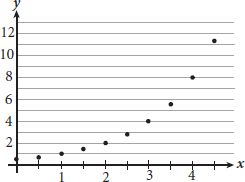Which of the following equations best models the data shown in the scatterplot above?

• A.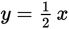• B.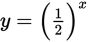• C.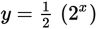• D.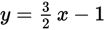Explanation:

C

Difficulty: Medium

Category: Problem Solving and Data Analysis / Scatterplots

Strategic Advice: Whenever you need to match data to a model, use the trend (shape) of the data to determine the type of model (linear, exponential, quadratic, or polynomial), and then use specific data points (if needed) to identify the best choice.

Getting to the Answer: The trend of the data shown in the scatterplot is increasing, slowly at first and then much more quickly, which indicates an exponential model. This means you can eliminate A and D (both are linear). To choose between B and (C), choose a data point (preferably one that lies on a grid-line and represents whole number values) and test it in each answer choice. Avoid x = 0 and x = 1 because those numbers have special properties when exponents are involved. Using the point (3, 4), the results are: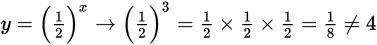Eliminate B, making (C) the correct choice. If you're still unsure, you can check (C):